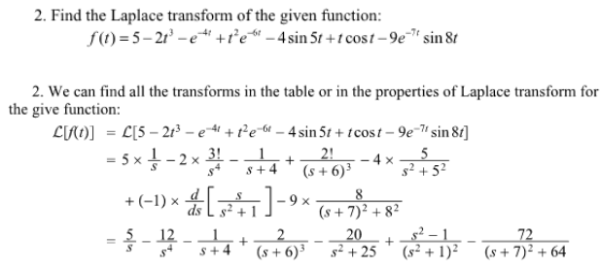Find the Laplace transform of the given function: f(t)=5-2t^3-e^(-4t)+t^2e^-6t-4sin5t+tcost-9e^-7tsin8t .Find the Laplace transform of the given function: f(t)=5-2t^3-e^(-4t)+t^2e^-6t-4sin5t+tcost-9e^-7tsin8t .

System Dynamics Page 2 dynamics dynamics dynamics dynamics dynamics dynamics dynamics System dynamics Page 3